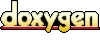# ACE_Based_Pointer_Basic< CONCRETE > Member List

This is the complete list of members for ACE_Based_Pointer_Basic< CONCRETE >, including all inherited members.
 ACE_ALLOC_HOOK_DECLARE ACE_Based_Pointer_Basic< CONCRETE > ACE_Based_Pointer_Basic(void) ACE_Based_Pointer_Basic< CONCRETE > ACE_Based_Pointer_Basic(CONCRETE *initial) ACE_Based_Pointer_Basic< CONCRETE > ACE_Based_Pointer_Basic(const ACE_Based_Pointer_Basic< CONCRETE > &) ACE_Based_Pointer_Basic< CONCRETE > ACE_Based_Pointer_Basic(const void *base_addr, int o) ACE_Based_Pointer_Basic< CONCRETE > addr(void) const ACE_Based_Pointer_Basic< CONCRETE > base_offset_ ACE_Based_Pointer_Basic< CONCRETE > ` [protected]` dump(void) const ACE_Based_Pointer_Basic< CONCRETE > operator *(void) const ACE_Based_Pointer_Basic< CONCRETE > operator CONCRETE *() const ACE_Based_Pointer_Basic< CONCRETE > operator!=(const ACE_Based_Pointer_Basic< CONCRETE > &) const ACE_Based_Pointer_Basic< CONCRETE > operator+=(int index) ACE_Based_Pointer_Basic< CONCRETE > operator<(const ACE_Based_Pointer_Basic< CONCRETE > &) const ACE_Based_Pointer_Basic< CONCRETE > operator<=(const ACE_Based_Pointer_Basic< CONCRETE > &) const ACE_Based_Pointer_Basic< CONCRETE > operator=(CONCRETE *from) ACE_Based_Pointer_Basic< CONCRETE > operator=(const ACE_Based_Pointer_Basic< CONCRETE > &) ACE_Based_Pointer_Basic< CONCRETE > operator==(const ACE_Based_Pointer_Basic< CONCRETE > &) const ACE_Based_Pointer_Basic< CONCRETE > operator>(const ACE_Based_Pointer_Basic< CONCRETE > &) const ACE_Based_Pointer_Basic< CONCRETE > operator>=(const ACE_Based_Pointer_Basic< CONCRETE > &) const ACE_Based_Pointer_Basic< CONCRETE > operator[](int index) const ACE_Based_Pointer_Basic< CONCRETE > target_ ACE_Based_Pointer_Basic< CONCRETE > ` [protected]`

Generated on Thu Nov 9 11:20:18 2006 for ACE by1.3.6